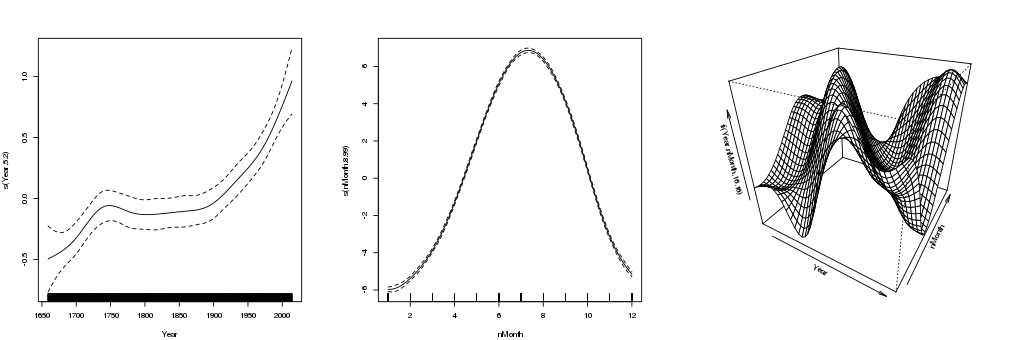[This article was first published on From the Bottom of the Heap - R, and kindly contributed to R-bloggers]. (You can report issue about the content on this page here)
Want to share your content on R-bloggers? click here if you have a blog, or here if you don't.

I ended the last post with some pretty plots of air temperature change within and between years in the Central England Temperature series. The elephant in the room1 at the end of that post was is the change in the within year (seasonal) effect over time statistically significant? This is the question I’ll try to answer, or at least show how to answer, now.

The model I fitted in the last post was

[ y = _0 + f(x_1, x_2) + , N(0, ^2) ]

and allowed, as we saw, for the within year spline/effect to vary smoothly with the trend or between year effect. Answering our scientific question require that we determine whether the spline interaction model (above) fits the data significantly better than the additive model

[ y = 0 + f{}(x_1) + f_{}(x_2) + , N(0, ^2) ]

which has a fixed seasonal effect?

The model we ended up with was the spline interaction with an AR(7) in the residuals. To catch you up, the chunk below loads the CET data and fits the model we were left with at the end of the previous post

To answer our question, we want to fit the following two pseudo-code models and compare them using a likelihood ratio test

As is often the case in the real world, things aren’t quite so simple; there are several issues we need to take care of if we are going to really be testing nested models and the smooth terms that we’re interested in, specifically we need to

1. ensure that the models really are nested models,
2. fit using maximum likelihood (`method = “ML”`) not residual maximum likelihood (`method = “ML”`) because the two models have different fixed effects
3. fit the same AR(7) process in the residuals in both models.

To compare additive models we really want to ensure that the fixed effects parts are properly nested and appropriate for an ANOVA-like decomposition of main effects and interactions. mgcv provides a very simple way to achieve this via a tensor product interaction smooth and the `ti()` function. `ti()` smooths are created in the same way as the `te()` smooth we encountered in the last post, but unlike `te()`, `ti()` smooths do not incorporate the main effects of the terms involved in the smooth. It is further assumed therefore that you have included the main effects smooths in the model formula.

Hence we can now fit models like

and be certain that the `s(x1)` and `s(x2)` terms in each model are equivalent. Note that you can use `s()` or `ti()` for these main effects components; if you have a single variable involved in a `ti()` term you get the main effect. I’m going to use `s()` in the code below, because I had better experience fitting the `gamm()` models we’re using with `s()` rather than `ti()` main effects.

Fitting with maximum likelihood instead of residual maximum likelihood is just a simple matter of using `method = “ML”` in the `gamm()` call.

The last thing we need to fix before we proceed is making sure that the main effects model and the main effects plus interaction model both incorporate the same AR(7) process that we fitted originally and which we refitted here earlier as `m`. To achieve this, we need to supply the AR coefficients to `corARMA()` when fitting our decomposed models, and indicate that `gamm()` (well, the underlying `lme()` code) shouldn’t try to estimate any of the parameters for the AR(7) process.

We can access the AR coefficients of `m` through the `intervals()` extractor functions and a little bit of digging. In the chunk below I store the AR(7) coefficients in the object `phi`. Now when fitting the `gamm()` models we have to pass `value = phi, fixed = TRUE` to the `corARMA()` bits of the model call to have it use the supplied coefficients instead of estimating a new set.

We are now ready to fit our two models to test whether the interaction smooth is required

The `anova()` method is used to compared the fitted models

There is clear support for `m1` the model that allows for the seasonal smooth to vary as a smooth function of the trend over the model with additive effects.

What does our model say about the change in monthly temperature over the past century? Below I simply predict the temperature for each month in 1914 and 2014 and then compute the difference between years.

A plot of the temperature differences2 is shown below, being produced by the following code

Most months have seen at least ~0.5°C increase in mean temperature between 1914 and 2014, with October and November both experiencing over a degree of warming over the period.

Before I finish, it is instructive to look at what the `ti()` term in the decomposed model looks like and representsSmooths for the spline interaction model including a tensor product interaction smooth

The first two terms are the overall trend and seasonal cycle respectively. The third term, shown as a perspective plot, is the tensor production interaction term. This term reflects the amount by which the fitted temperature is adjusted from the overall trend and seasonal cycle for any combination of month and year.

1. well, one of the elephants; I also wasn’t happy with the AR(7) for the residuals

2. If I was being more thorough, I could use the prediction matrix feature of `gam()` models to put approximate confidence intervals on these differences.